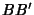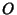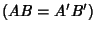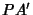## Concentric Circles

The region between two Concentric circles of different Radii is called an Annulus.

Given two concentric circles with Radiiand, what is the probability that a chord chosen at random from the outer circle will cut across the inner circle? Depending on how the random'' Chord is chosen, 1/2, 1/3, or 1/4 could all be correct answers.

1. Picking any two points on the outer circle and connecting them gives 1/3.

2. Picking any random point on a diagonal and then picking the Chord that perpendicularly bisects it gives 1/2.

3. Picking any point on the large circle, drawing a line to the center, and then drawing the perpendicularly bisected Chord gives 1/4.
So some care is obviously needed in specifying what is meant by random'' in this problem.

Given an arbitrary Chordto the larger of two concentric Circles centered on, the distance between inner and outer intersections is equal on both sides. To prove this, take the Perpendicular topassing throughand crossing at. By symmetry, it must be true thatandare equal. Similarly,andmust be equal. Therefore,equals. Incidentally, this is also true for Homeoids, but the proof is nontrivial.